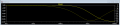# Bass Boost in-Line circuit between headphone and amp

#### sam68

Joined Mar 2, 2017
41
I need help understanding out to implement this circuit. Will it work if I place it between a laptop headphone jack and my small “Pam xxx” mono amp. It is suppose to attenuate the treble so the bass will be “boosted.” See figure 3 in the uploaded file. Questions:
1. The left “IN” and left “Grn” are from my laptop headphone jack, correct?
2. I assume that the arrow for the Right “out” is from the pot to the amp as well as the right ground is connected to the left ground and then to the amp, correct?

I would like to change the values but I don't know how: The current components have a treble reduction range between 0 and 6 db but I would like for someone to tell me what new component value for the pot and for the resistor is needed to make the range 0 to 12db.

Maybe the explanation below (not mine) can help you but I don’t understand how to change those values. I assume that after I change the values to go from 0 to 12db, that the C1 value formula (starting HZ) will be the same or does that need to be changed too?.

Thanks

scott

Variable Equalizer
The value of C1 is determined by the following (1/2 of the pot value is added to the resistor value, since both are in series, and a typical situation will have a pot setting of around halfway (note = that changes the starting frequency to begin the attenuation, ie 383 hz)

C1 = 1 / 2 * * (R1 + (VR1 / 2)) * f so an example = C1 = 1 / 2 * * 15k * 383 = 27nF

The only change that you will need is to the value of C1 according to the above formula.)

It is essential that the compensation circuit be driven from a low impedance source, and the load impedance should be reasonably high. There will be little error with loading above 20k, but basically the higher the impedance, the better. Opamp buffers at the input and output may be used if you cannot ensure that the source impedance is 100 ohms or less, and that the load impedance of the following stage is greater than 20k. My recommendation would be to use a buffer stage at the output with an input impedance of about 100k.

#### sam68

Joined Mar 2, 2017
41
I would appreciate if someone could help me. I posted the explanation and attached a picture of the circuit but I have the questions at the top of the post.
thanks

#### Alec_t

Joined Sep 17, 2013
13,043
Q1. Yes.
Q2. Yes.
Reduce R1 to 3.3k to get ~12dB reduction. Keep the same pot value.

Last edited:

#### sam68

Joined Mar 2, 2017
41
Hey Alec,
Thanks for your response.
1. For this circuit, at what ohm level is considered "high amp input impedance?"
2. Low source impedance would be under 100 ohms, (ie headphone or aux out,) correct?
3. If I wanted to change the -12db to -9db, then the resister would be around 6.5?
4. I don't know too much about how capacitors would be used to change the frequency where the baffle step would start and end but I would like to have it start at 800 hz (0 db reduction) and have the shelf end at around 1600 hz (one octave) and continue the shelf at (-9 or -12 to the end of the treble range.) Do you have an idea what value of capacitor is needed? Would it be non-polarized and are electrolytics OK?
Thanks again,
scott

#### Alec_t

Joined Sep 17, 2013
13,043
1) At least ~10 times the circuit's impedances, so I'd suggest at least 100k for this circuit.
2) Less than ~ 1/10 of the circuit's impedances, so headphone output would be ok.
3) Try 5.6k or 6.8k. You won't hear much/any difference.
4) Such a simple circuit can't give a 0dB to -9 or -12 dB drop off over the 800-1600Hz range while plateauing below 800Hz and above 1600Hz. The best you can do is probably use a 18nF non-polarised cap to give a response like this :-